# 主要贡献

• 提出了一种新颖的对偶注意力网络，利用自注意力机制提高特征表示的判别性。
• 位置注意力模块用于学习特征的空间依赖性，通道注意力模块用来学习通道之间的内部关联性。
• 在cityscapes，PASCAL context，COCO stuff上实现了更好的性能

# 方法backbone是ResNet，50或者101，都行，重点是融合空洞卷积核并删除了池化层的ResNet，其实就是现在流行的deeplab中使用的resnet结构了，注意resnet输出的特征图是原始尺寸的$\frac{1}{8}$倍。

## 位置注意力模块 PAM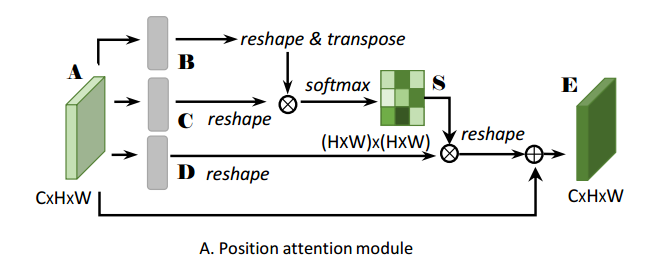A就是模块的输入，它被分别送到三个卷积层中获得了B，C，D。这四个特征图都是一样大小的，都是$R^{C\times H\times W}的$。现在把B和C都reshape为$R^{C \times N}$，其中$N= H\times W$ 。 然后将C的转置和B相乘，得到了$S \in R^{N \times N}$，然后按列做softmax运算。见原文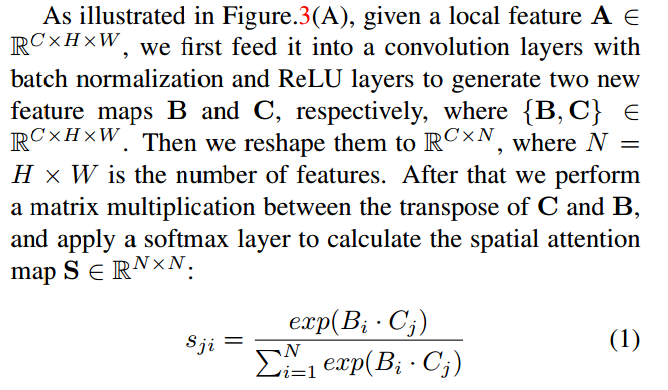$s_{ji}$就是第j行第i列的元素，它等于$B$的第i行乘上$C$的第J列的指数除以下面那串玩意，如果你耐心推到，就是把得到的S按列求softmax运算，再赋值给S。$s_{ji}$代表着第i个位置的特征对第J个位置的影响，如果这两个位置恰好都是属于同一类的像素点，那么$s_{ji}$就会产生很大的值，这样就会突出相似特征之间的联系，但其实为啥能这样我没看懂。再说一下，$B_i,B_j$其实都是$R^{1\times C}$的，i和j都是遍历$1,2,...,H\times W$的，其实是空间维度上的运算，空间维度上的一个像素点的特征不就是$R^{1\times C}$的嘛！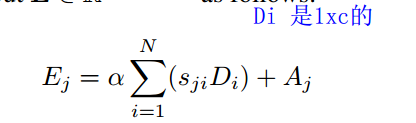$D_i$$D$的第$i$列。a是训练参数，也是自注意力参数，等下说。按上面的公式，J遍历到$1,2,...,C$就得到了$E \in R^{C\times N}$$R^{C\times H \times W}$。之后这个特征图在经过一个卷积层，论文中没写（源码中写了），作为这个模块的输出。

## 通道注意力模块 CAM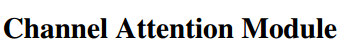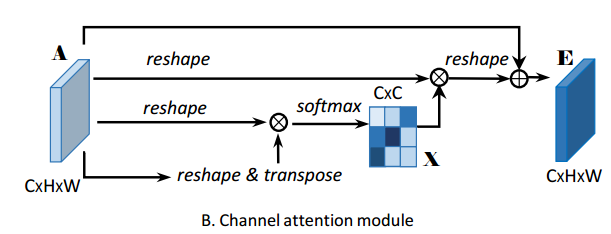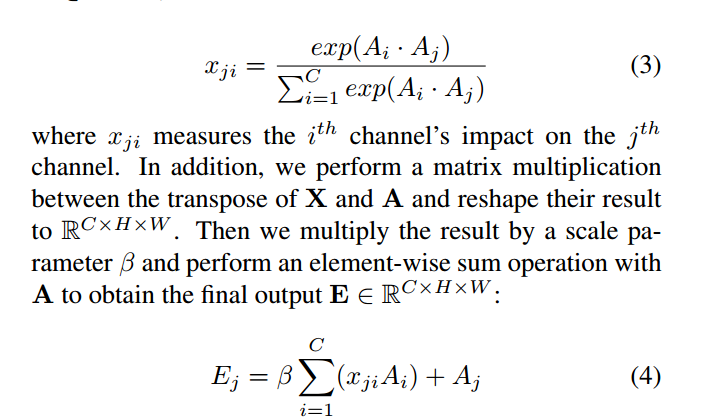# 实验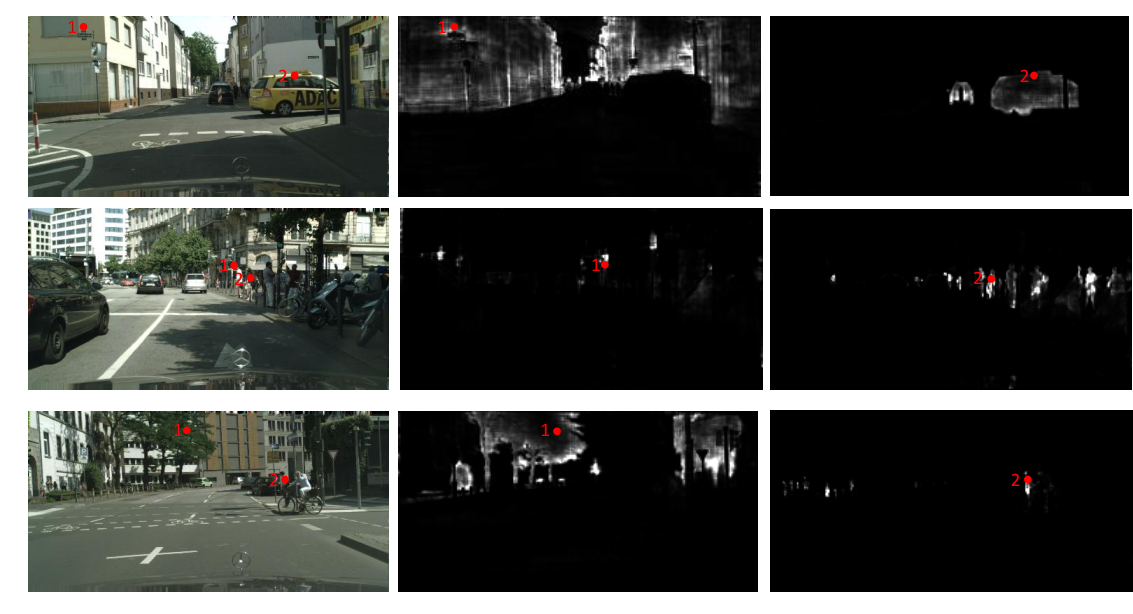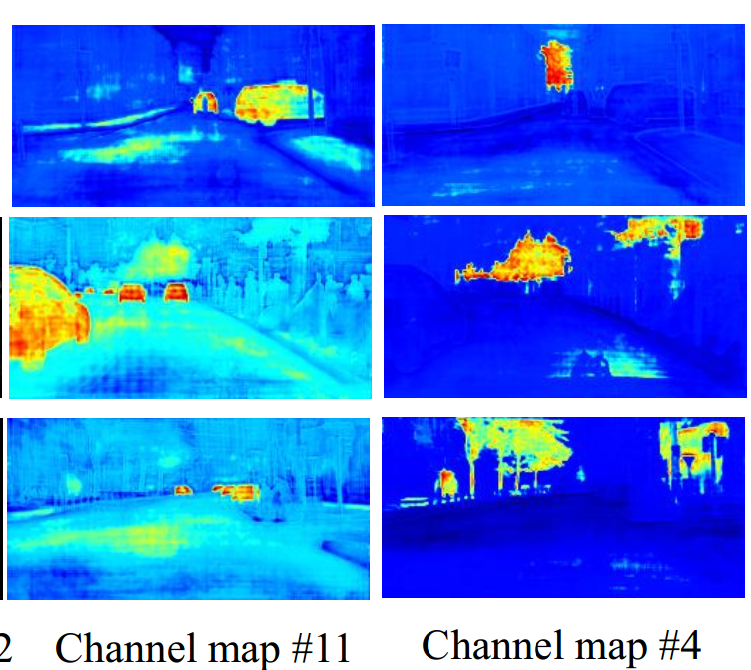# 从源码看PAM和CAM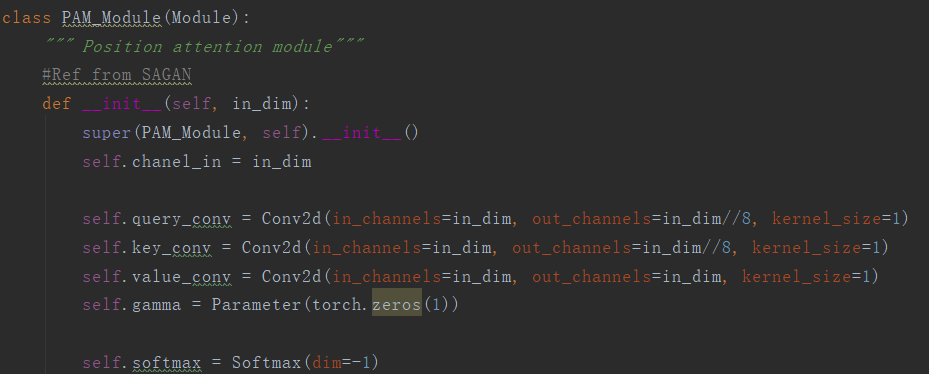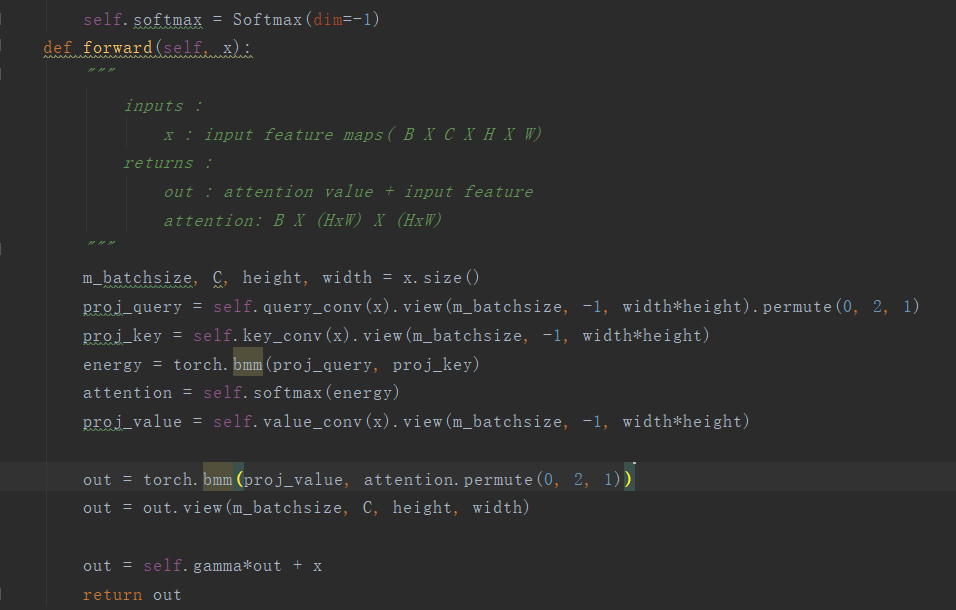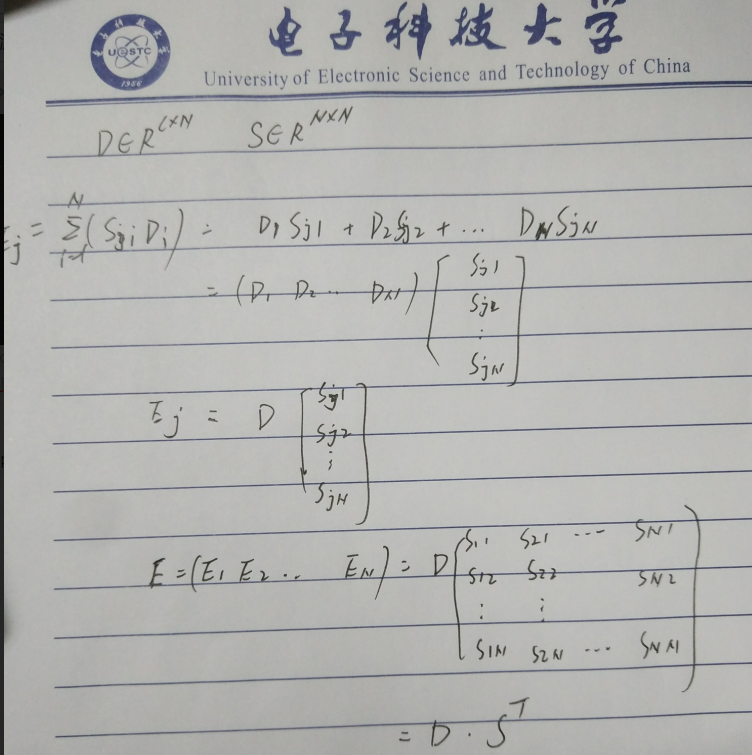out = torch.bmm(proj_value, attention.permute(0, 2, 1))  # permute 就是转置的意思
out = out.view(m_batchsize, C, height, width)

out = self.gamma*out + x


CAM 也是一样差不多的。就跳过了。那么既然官方开源了代码，我当然要试试看看啦

# 实现效果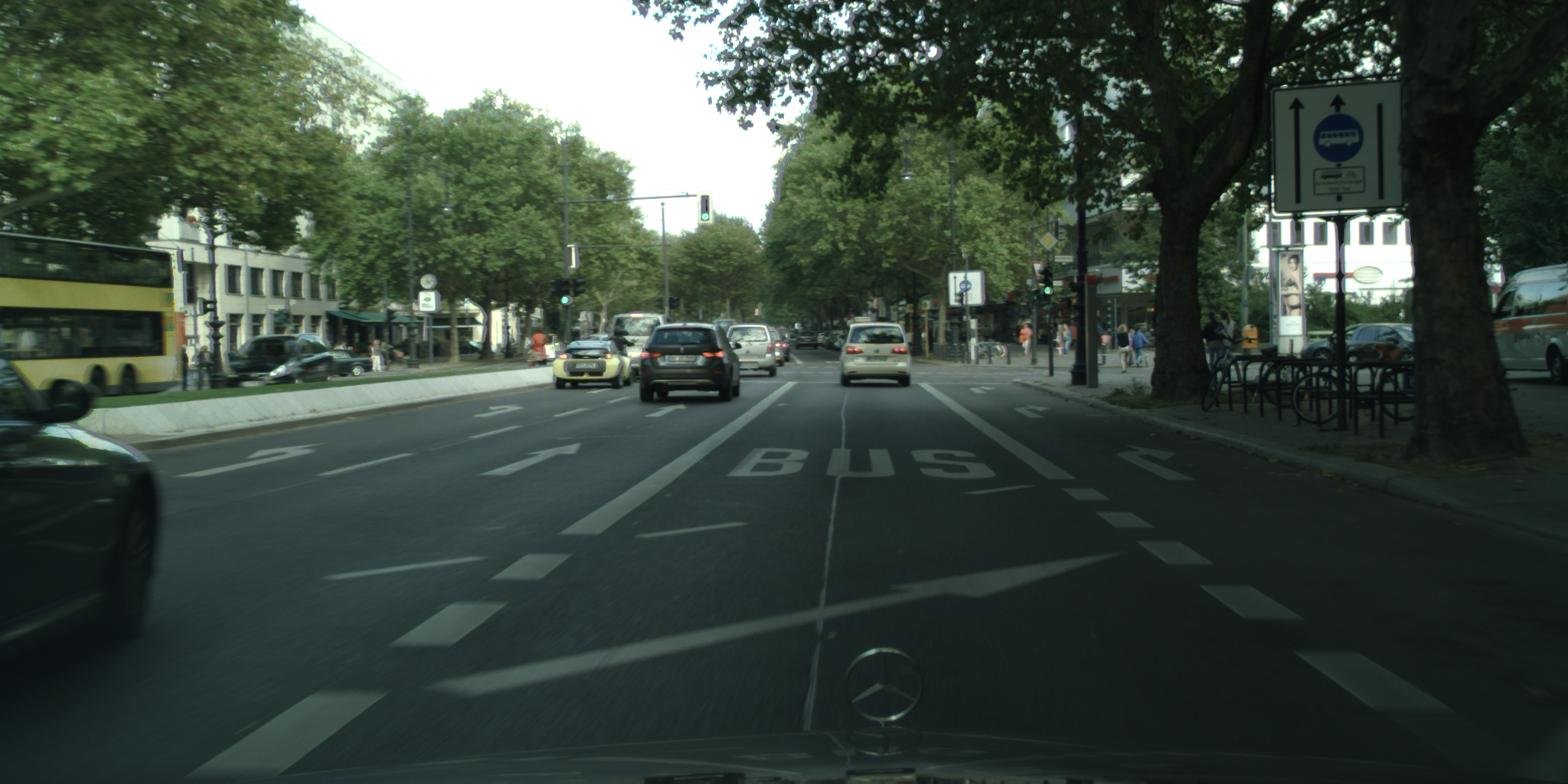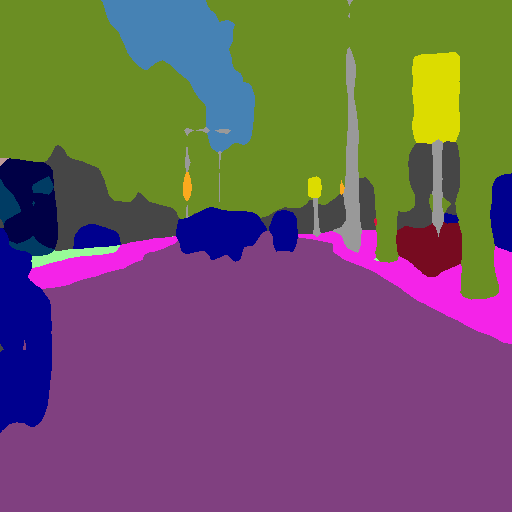上面的结果是我直接将2048x1024大小的原图resize成512x512的大小的结果，所以图像有不成比例的扭曲。因为内存占用太大了。如果我想获得好的结果，同样可以crop在将patch拼接，我懒就没弄了。但就从上面的结果来看，还是不错的，毕竟用的也是人家做实验的预训练模型。

;